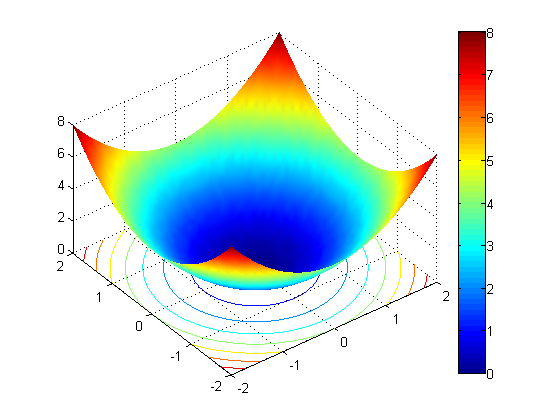# HELP – I Got the Wrong Answer for My Optimization!

How often I have heard this!?! It turns out there are a bunch of reasons why you might not get the answer you expect when performing an optimization task. These possibilities include the answer not being unique, the optimization method used isn't global and therefore returns a local optimal solution, and the problem, as stated, isn't the problem you really want to solve. Let me tell you more about a recent case.

### What We Heard

We heard from a user that fmincon, from Optimization Toolbox, gets the wrong answer for the problem posed in myOptFun. In fact, the optimization gets stuck at the initial point.

### The Function

Let's take a quick look at the function. The user told us that it was a simple paraboloid of revolution. I'll calculate z values on a grid and display as a surface.

[xx,yy] = meshgrid(-2:.1:2);
zz = xx.^2+yy.^2;
surfc(xx,yy,zz)
colorbar
view(-37.5,50)### Run the Example

First I do some housekeeping.

opt = optimset('display', 'final');
warning off optim:fmincon:SwitchingToMediumScale

Next, I'll run the example using the user's objective function.

lb = [-5;-5];
ub = [5;5];
x0 = [1;1];
[x fval exitflag output lamb grad hess] = ...
fmincon(@myOptFun,x0,[],[],[],[],lb,ub,[],opt);
Optimization terminated: first-order optimality measure less
than options.TolFun and maximum constraint violation is less
than options.TolCon.
No active inequalities.


According the user, the optimal answer is 0 and can be found at (0,0). Instead, look what we find.

x
x =
1
1

fval
fval =
2


### Why Did the Optimization Not Find Zero?

The source of the confusion is in myOptFun. Now let me show you the code.

type myOptFun
function f = myOptFun(X)
formule = 'x.*x+y.*y';
formule = strrep(formule,'x',num2str(X(1)));
formule = strrep(formule,'y',num2str(X(2)));
f = eval(formule);


It's the use of num2str specifically. As the documentation says, the default format for num2str is '%11.4g' which means I am calculating results to 4 significant figures. Calling num2str(1), the result is 1 and the same is true for num2str(1+1e-5). In other words the accuracy of the customer's objective function cannot be expected to be better than 1e-4. However, the default tolerances for TolFun and TolX are 1e-6. More importantly DiffMinChange ("minimum change in variables for finite difference derivatives") is 1e-8. When the first finite difference calculations are done, the approximate first-order derivatives of the customer's objective function are zero and fmincon stops.

### How to Fix the Optimization

I have several options for obtaining a "better" result. I can

• increase the accuracy of the objective function
• adjust the relevant tolerances to be larger to accomodate the resolution of the objective function
• provide exact first-order derivatives (TolFun and TolX still need to be less than 1e-4).

### Change the Objective Function

Let's try the same optimization with a modified objective function.

[xFixed fvalFixed exitflag output lamb grad hess] = ...
fmincon(@myOptFunFixed,x0,[],[],[],[],lb,ub,[],opt);
Optimization terminated: first-order optimality measure less
than options.TolFun and maximum constraint violation is less
than options.TolCon.
No active inequalities.

xFixed
xFixed =
1.0e-015 *
0.4441
-0.2220

fvalFixed
fvalFixed =
2.4652e-031


### Have You Hit a Similar Snag?

If you have hit a similar snag, it would be great if you could share it here.

Published with MATLAB® 7.6

|